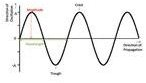# Physical Science Lesson Plans: Wave Motion Lesson

Page content

## An Introduction to Waves

To introduce your students to the concept of waves, conduct the following activities in your class.

Activity 1: Drop a pebble into a tank of still water (or any large and fairly deep vessel). What happens?

The surface of the water gets disturbed and it travels outward from the point of disturbance. This is a wave. Do the particles at the point of disturbance move outward too?

Activity 2: Put some cork pieces on the disturbed surface.

Observe that the cork pieces move up and down but do not move away from the center of the disturbance.

A disturbance travels from one point to another point away from the source, but there is no transport of material or medium itself.

## Transverse and Longitudinal Waves

In the light of the definition of a wave, explain the two directions involved in the study of wave motion namely:1. The direction of propagation of wave, that is, the direction in which the disturbance travels.

2. The disturbance is transferred due to the oscillation of the particles of the medium involved. The direction of these oscillations is the second direction.

Show this link on wave motion from Pennsylvania State University to your class and ask them to identify these two directions in the case of transverse and longitudinal waves shown on the page.

Hence introduce and define the two kind of waves, namely,

Transverse Waves : When these two directions are perpendicular to each other.

Longitudinal Waves : When these two directions are parallel.

## Properties of Waves

• Draw the above diagram for explaining transverse waves.

• For the case of longitudinal waves, draw a similar diagram, only the crests will be rarefactions and troughs will be compressions in this case.

Students should understand these points regarding the graphs:

1. The direction of oscillation has been shown on an axis perpendicular to the direction of propagation. However, the graph does not mean that these directions are perpendicular in both these cases.

2. On the graph of transverse motion, the y-axis shows “the displacement of the medium particles” in the y direction, from their equilibrium position whereas on the graph of longitudinal motion, “the displacement of the medium particles” from their equilibrium position in the x direction itself is plotted on the y-axis.

Refer to the above image to define the terms amplitude(A), Wavelength(λ), time period(T), frequency(f), time period(T) and velocity (v), stressing on the bolded keywords. If Simple Harmonic Motion has been taught before, then some of these terms will be a review to the students.

Amplitude (A) : The maximum displacement of the oscillating medium particles from the original position. Explain the terms Crests, Troughs, Rarefactions and Compressions. The units are that of distance.

Phase of Oscillation : Two particles are said to be in the same phase of oscillation when they are equally displaced from the original position and are moving in the same direction. The units are that of angle.

Wavelength (λ)****: The distance (measured in the direction of propagation of wave) between points of two points in the same phase of oscillation. The units are that of distance. Commonly Å(1 Angstrom is 10-10 meters)

Frequency (f) : The number of complete oscillations in a second made by a particle about the equilibrium position. It is quoted in hertz(Hz).

Time Period (T) : The time required for one complete oscillation of a particle is the time period. It is the reciprocal of frequency.

Velocity of a Wave (v) : The speed with which wave travels in a medium.

## Velocity of a Wave

Introduce your students to the formula of the velocity of a wave:

velocity = frequency X wavelength

or, v = f.λ

Here, f is the frequency of the wave, λ is the wavelength and v is the velocity of the wave.

The velocity of the wave is a function of the medium alone. The wavelength and frequency are functions of the source. Hence, by changing the frequency of a wave, its wavelength will be changed, but its speed cannot be altered if the medium is kept the same.

## This post is part of the series: Waves and Sound

This series covers lesson plans covering the concepts of wave motion and sound.